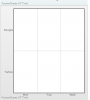## Recommended Posts

Hello,

Here is xml:

<chart><dataset><set rowId='Google' columnId='Mon' value='68'/> <set rowId='Google' columnId='Tue' value='35'/><set rowId='Google' columnId='Wed' value='95'/><set rowId='Yahoo' columnId='Mon' value='0'/> <set rowId='Yahoo' columnId='Tue' value='71'/></dataset></chart>

Attached is the screen shot. (Values and background color not coming)

If possible, give me a demo.

Thanks,

RohitEdited by rohit111111

##### Share on other sites

Hello,

Here is xml:

<chart><dataset><set rowId='Google' columnId='Mon' value='68'/> <set rowId='Google' columnId='Tue' value='35'/><set rowId='Google' columnId='Wed' value='95'/><set rowId='Yahoo' columnId='Mon' value='0'/> <set rowId='Yahoo' columnId='Tue' value='71'/></dataset></chart>

Attached is the screen shot. (Values and background color not coming)

If possible, give me a demo.

Thanks,

Rohit

Hi,

Thanks for the mail.

Please try providing a correct format with the <colorRange> to render the chart correctly.

Ex:

```<chart>
<dataset>
<set rowId='Yahoo' columnId='Mon' value='0'/>
<set rowId='Yahoo' columnId='Tue' value='71'/>
</dataset>

<colorRange mapbypercent='1' gradient='1' minValue='0' code='00CCFF' startLabel='Poor' endLabel='Good'>
<color code ='F2CF63' maxValue='70' label='AVERAGE'/>
<color code ='D99036' maxValue='100' />

</colorRange>

</chart>```

Hope this helps.##### Share on other sites

Thanks.

Edited by rohit111111